Many people know that you can use "WHERE processing" in SAS to filter observations. A typical use is to process only observations that match some criterion. For example, the following WHERE statement processes only observations for male patients who have high blood pressure:

WHERE Sex='Male' & Systolic > 140;


In this statement, a character variable (SEX) is tested for equality with a specified value, and a numerical variable (SYSTOLIC) is tested for inequality. Most people know that you can use operators (such as '=' and '>') to filter observations, but did you know that SAS also supports using functions as part of a WHERE statement? This article gives a few quick examples and discusses a neat trick that you can use when you want to filter observations, but you cannot use a WHERE statement directly.

### Where can you use WHERE?

In SAS, there are four ways to perform WHERE processing:

• The WHERE= data set option: This option is places after the name of the data set when you use the SET statement the DATA step or the DATA= option in a procedure. The WHERE= option reads only the observations that satisfy the criteria.
• The WHERE statement: This global statement filters observations that have been read into the DATA step or procedure. The WHERE statement filters those observations. Only the observations that satisfy the criteria are processed by the procedure.
• In a WHERE clause in the SQL procedure or in PROC IML. A WHERE clause might not support the full range of operators and functions that are supported in the WHERE statement.
• Use a DATA step view to create an indicator variable. In a subsequent call to a procedure, use a WHERE statement to process observations for which the indicator variable has a specific value. Technically, this is not truly WHERE-processing, but it is a very useful technique, so I want to include it in this list.

The WHERE statement and the WHERE= data set option support similar options. The examples in this article use the WHERE statement, but the examples work equally well if you use the WHERE= data set option. For brevity, I will primarily refer to "the WHERE statement" and will show only one example that uses the WHERE= data set option.

The SAS documentation includes a chapter about WHERE-expression processing, which I will refer to as "WHERE processing." The doc spends many pages describing many special-purpose operators that you can use in WHERE statements. However, it only briefly mentions that you can use many function calls as well.

### Using operators and functions in a WHERE statement

Before discussing functions, I want to point out that you can use arithmetic operators: plus, minus, multiplication, and so forth) in a WHERE statement. For example, the following statements use arithmetic operators to include only certain observations:

data Have2; set sashelp.heart; where Systolic - Diastolic > 60 /* wide pulse pressure */ & weight / height**2 * 703 > 25; /* BMI > 25 */ run;

The resulting data set contains only observations (patients in a heart study) for which the difference between the systolic and diastolic blood pressure is large, and for which the patient's body-mass index is also large.

The previous statements do not call any SAS functions in the WHERE statement. The most popular functions are probably string-manipulation functions such as the SUBSTR and SCAN functions. These functions enable you to filter observations according to the value of one or more character variables. The following example uses the UPCASE and SUBSTR functions to test whether the first two characters of the SEX variable indicate that the patient is male. The function calls will match strings like 'male', 'Male', and 'MALE'. For fun, I also show that you can use the SQRT function, which performs a square-root operation.

data Have3; set sashelp.heart; where upcase(substr(Sex, 1, 2))='MA' /* match first two characters of 'male' and 'Male' */ & sqrt(weight*703) / height > 5; /* alternative way to specify BMI */ run;

Another popular character function is the SCAN function. The following example uses the WHERE= data set option to read only the observations for which the cause of death includes the word 'Disease' as the third word. Matching values include "Cerebral Vascular Disease" and "Coronary Heart Disease":

/* an example that uses the WHERE= data set option */ data Have4; set sashelp.heart( where=(scan(DeathCause, 3) = 'Disease') ); run;

As mentioned earlier, the WHERE= data set option can be more efficient that the WHERE statement. The syntax requires additional parentheses but is otherwise similar to the WHERE statement.

### WHERE clauses in SAS IML

A customer recently noticed that the WHERE clause in SAS IML supports only a small subset of the operators that the WHERE statement supports. Furthermore, it does not support calling functions like the SUBSTR or SQRT functions. If you look at the documentation for the WHERE clause, you will notice that the syntax is of the form
Variable operator Value
which means that you can only use variable names on the left side of the WHERE clause. You cannot use arithmetic operations on variables or functions of variables. The following PROC IML statements show a valid syntax for a WHERE clause on the USE statement in PROC IML. An invalid syntax is shown in the comment:

proc iml; /* this WHERE clause works */ use sashelp.heart where( Sex='Male' & Systolic > 140 ); read all var {Systolic Diastolic}; close;   /* The following statement is NOT supported in the WHERE clause: USE sashelp.heart WHERE(Systolic - Diastolic > 60 & weight / height**2 * 703 > 25); */

Although the WHERE clause on the USE statement does not support expressions that use arithmetic operations or function calls, there is a simple trick that enables you to use the power of the WHERE statement in the DATA step to filter data as you read observations into IML. The trick is to use the SUBMIT/ENDSUBMIT block to create a DATA step that filters the data. For example, the following statements create a DATA step view. The view is read by the USE and READ statements in the IML language. It filters the data at runtime while the observations are read:

submit; data _Filter / view=_Filter; set sashelp.heart; where upcase(substr(Sex, 1, 2))='MA' & sqrt(weight*703) / height > 5; run; endsubmit;   use _Filter; read all var {Systolic Diastolic}; close;

There might be times in which you want to subdivide data into two or more subsets but only process one of the subsets. In that case, you can use a DATA step view to create an indicator variable. You can then use the indicator variable in a WHERE clause in IML to read the data. For example, the following statements create a view that contains a binary indicator variable named _INCLUDE. The WHERE clause on the USE statement reads the observations for which _INCLUDE=1:

submit; data _Filter / view=_Filter; set sashelp.heart; _Include = (upcase(substr(Sex, 1, 2))='MA' & sqrt(weight*703) / height > 5); run; endsubmit;   use _Filter WHERE(_Include=1); read all var {Systolic Diastolic}; close;

### Summary

This article serves as a reminder that the WHERE statement in SAS supports arithmetic operators and function calls, which means that you can use the WHERE statement to create sophisticated filters for your data. The WHERE= data set option is similar. However, some special-purpose languages in SAS support WHERE clauses that are more limited in their syntax. However, you can always use a DATA step view to filter data or to create an indicator variable that can be processed anywhere in SAS.

The post Use functions in a WHERE statement to filter observations appeared first on The DO Loop.

A previous article shows how to compute the probability density function (PDF) for the multivariate normal distribution. In a similar way, you can compute the density function for the multivariate t distribution. This article discusses the density function for the multivariate t distribution, shows how to compute it, and visualizes the density for a bivariate distribution in two different ways.

As for all multivariate computations, the computations are simpler when you express the formulas in terms of matrices and vectors. This article uses the SAS/IML language to evaluate the multivariate t distribution.

### A comparison of the normal and t distributions

Before discussing multivariate distributions, let's recall the univariate t distribution and compare its shape to the standard normal distribution. The density of the univariate t distribution with $\nu$ degrees of freedom has the following formula:
$f(x)=\frac { \Gamma((\nu +1)/2) } { \Gamma(\nu/2) \sqrt{\nu \pi} } \left(1+{\frac {x^{2}}{\nu }}\right)^{-(\nu +1)/2}$
Here, $\Gamma(\cdot)$ is the gamma function.

The t distribution is symmetric and converges to the standard normal distribution as $\nu \to \infty$. For any finite value of $\nu$, the t density function has more weight in the tails and, consequently, less weight near the center of the distribution. The following SAS DATA step uses the PDF function to compute the density function for the standard normal distribution and the t distribution with $\nu=10$ degrees of freedom. The call to PROC SGPLOT overlays the two density curves:

/* compare the univariate normal and t density curves (DF=10) */ data Compare; DF = 10; mu = 0; sigma = 1; do x = -4 to 4 by 0.1; Normal = pdf("Normal", x, mu, sigma); T = pdf("t", x, DF); output; end; run;   title "Comparison of Normal and t Distributions"; proc sgplot data=Compare; series x=x y=Normal; series x=x y=T / lineattrs=(thickness=2); run;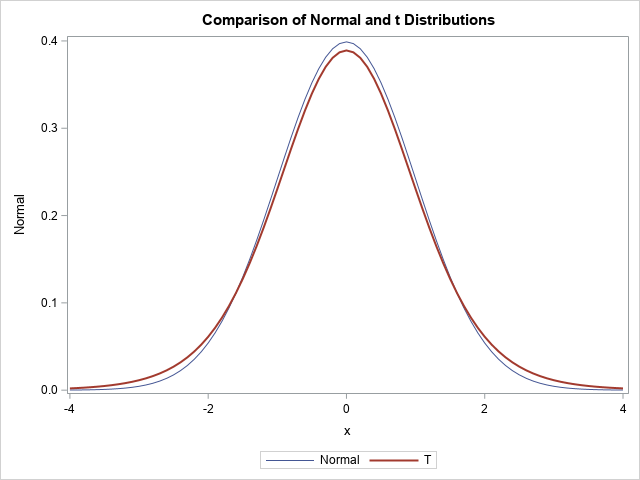As expected, the density curve for the t distribution is lower at the origin and higher in the tails, as compared with the standard normal distribution. This relationship is also true for the multivariate distributions: the multivariate t distribution looks a lot like the multivariate normal distribution but has less density near the center of the distribution and more density in the tails. In terms of a random sample, this means that data drawn from a multivariate t distribution has a wider spread: fewer points are near the center and more points are away from the center, as shown in the next section.

### Empirical density of the bivariate t distribution

The SAS/IML language supports random sampling from the multivariate normal and t distributions. You can use the RANDNORMAL function to sample from a multivariate normal distribution. In dimension p, the parameters are μ and Σ. The μ parameter is a mean vector of length p. The Σ parameter is a p x p covariance matrix. The multivariate t distribution also uses those parameters, as well as a scalar parameter that represents the degrees-of-freedom. The following SAS/IML program simulates 1,000 random observations from the bivariate normal and bivariate t (DF=10) distributions. The observations are written to a SAS data set, and PROC KDE is used to visualize the density of the bivariate scatter plot:

proc iml; call randseed(123); N = 1000; mu = {1 2}; Sigma = {2 1, /* correlation coefficient is sqrt(2) */ 1 1}; DF = 10; Z = randnormal(N, mu, Sigma); /* N obs from the MVN(mu, Sigma) distrib */ T = randmvt(N, DF, mu, Sigma); /* N obs from the MV t(DF) distrib */   A = Z || T; create Sample from A[c={z1 z2 t1 t2}]; append from A; close; QUIT;   /* Use PROC KDE to visualize bivariate density. See https://blogs.sas.com/content/iml/2019/09/18/visualize-density-bivariate-data.html */ %macro MakeDensityPlot(var1, var2); data Have; set Sample(keep=&var1 &var2); run; proc kde data=Have; bivar &var1 &var2 / bwm=1 ngrid=250 plots=none noprint; score data=Have out=Out(rename=(value1=&var1 value2=&var2)); run; proc sort data=Out; by density; run; proc sgplot data=Out; styleattrs wallcolor=verylightgray; scatter x=&var1 y=&var2 / colorresponse=density colormodel=ThreeColorRamp markerattrs=(symbol=CircleFilled) transparency=0.5; xaxis grid values=(-5 to 7); yaxis grid values=(-3 to 7); run; %mend;   title "Density of Multivariate Normal Sample"; %MakeDensityPlot(z1, z2) title "Density of Multivariate t Sample (DF=10)"; %MakeDensityPlot(t1, t2)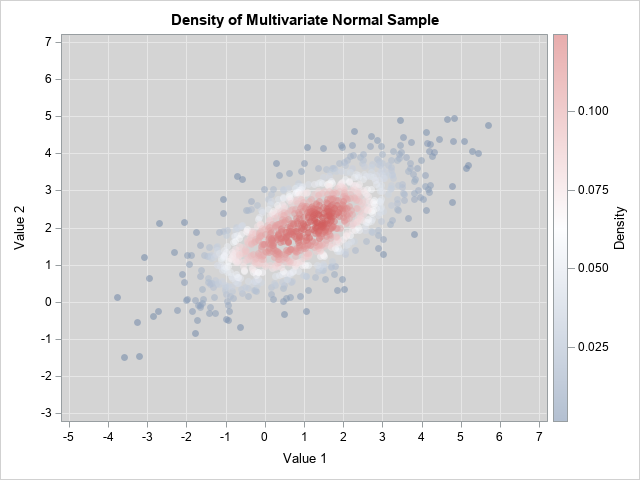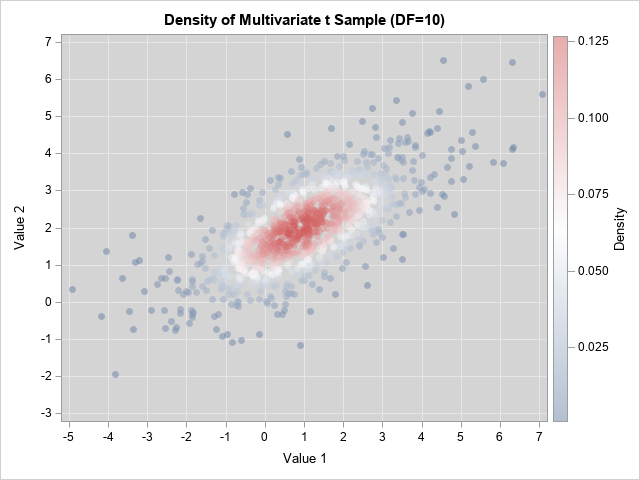The graphs are both scatter plots, but the observations have been colored according to an estimate of the bivariate density. You can see that the points for the bivariate normal distribution are more concentrated near the origin (the center of these data) and have less variation compared to the points for the bivariate t distribution. In both cases, the Σ parameter provides a way to model the correlation between the variables.

These graphs show the empirical density for a random sample. For very large samples, the empirical density should be close to the probability density, which is presented in the next section.

### Probability density for the multivariate t distribution

The standard formula for the univariate t distribution does not have a location or scale parameter, but you can add those parameters so that the t distribution is analogous to the univariate normal distribution. In the earlier DATA step, you could modify the PDF by using the expression
T = 1/sigma * pdf("t", (x-mu)/sigma, DF);

In a similar way, you can add location and covariance parameters to the multivariate t distribution. Recall that the multivariate normal PDF contains the expression d2=(x-μ)TΣ-1(x-μ), where d is the Mahalanobis distance between the multidimensional vector x and the mean vector μ. The same expression appears in the following formula for the density of the multivariate t distribution:
$f(x)=\frac {\Gamma ((\nu +p)/2) } {\Gamma (\nu/2) (\nu \pi)^{p/2} |\Sigma|^{1/2} } \left(1+ \frac{1}{\nu} (x - \mu)^T \Sigma^{-1} (x - \mu)\right)^{-(\nu +p)/2}$

The SAS/IML language supports the MAHALANOBIS function, which enables you to compute that quadratic term quickly and efficiently. The following SAS/IML module defines the PDF for the multivariate t distribution. For comparison, I also define the PDF for the multivariate normal distribution. I then evaluate the PDFs on a uniform bivariate grid of values and write the values to a data set. For later use, the program also evaluates the logarithm of the densities.

/* evaluate multivariate t density */ proc iml; /* Probability density for the MV normal distribution. See https://blogs.sas.com/content/iml/2012/07/05/compute-multivariate-normal-denstity.html */ start MVNormalPDF(x, mu, cov); p = nrow(cov); const = (2*constant("PI"))##(p/2) * sqrt(det(cov)); d = Mahalanobis(x, mu, cov); phi = exp( -0.5*d##2 ) / const; return( phi ); finish;   /* Probability density for the multivariate t distribution */ start MVTPDF(x, nu, mu, cov ); /* DF = nu degrees of freedom */ p = nrow(cov); pi = constant("PI"); np2 = (nu+p)/2; const = Gamma(np2) / (Gamma(nu/2) * (nu*pi)##(p/2) * sqrt(det(cov))); d = Mahalanobis(x, mu, cov); f = const * (1 + d##2/nu)##(-np2); return( f ); finish;   /* 2-D example */ mu = {1 2}; Sigma = {2 1, /* correlation coefficient is sqrt(2) */ 1 1};   xx = do(-5, 7, 0.2); yy = do(-3, 7, 0.2); xy = ExpandGrid(xx, yy);   NormalDensity = MVNormalPDF(xy, mu, Sigma); DF = 10; TDensity = MVTPDF(xy, DF, mu, Sigma);   logNormal = log10(NormalDensity); /* Optional: Compute LOG10 of densities */ logT = log(TDensity); x1 = xy[,1]; x2 = xy[,2]; create BivarDensity var {"x1" "x2" "NormalDensity" "TDensity" "logNormal" "logT"}; append; close; QUIT;

You can use a contour plot to visualize the bivariate normal a bivariate t densities:

/* Define and use the ContourPlotParm GTL template from https://blogs.sas.com/content/iml/2012/07/02/create-a-contour-plot-in-sas.html */ proc sgrender data=BivarDensity template=ContourPlotParm; dynamic _TITLE="Bivariate Normal Density, corr=sqrt(2)" _X="x1" _Y="x2" _Z="NormalDensity"; run;   proc sgrender data=BivarDensity template=ContourPlotParm; dynamic _TITLE="Bivariate t Density, corr=sqrt(2), DF=10" _X="x1" _Y="x2" _Z="TDensity"; run;I don't know about you, but I cannot see a difference between the contour plots on this scale. Recall that for the 1-D distributions, the density curves were very close to each other, so this isn't too much of a surprise. Both densities are concentrated near the mean parameter, which is (1, 2). Theoretically, the normal density has more mass near the mean.

To better compare the distribution, you can switch to the log scale, which will magnify the small differences between the densities. The following statements create contour plots of the logarithm of the densities. So that both plots use the same scale, I use only observations where the density is greater than 1E-6 or, equivalently, the base-10 logarithm is greater than -6.

proc sgrender data=BivarDensity(where=(LogNormal >= -6)) template=ContourPlotParm; dynamic _TITLE="Logarithm of the Bivariate Normal Density, corr=sqrt(2)" _X="x1" _Y="x2" _Z="logNormal"; run;   proc sgrender data=BivarDensity(where=(LogT >= -6)) template=ContourPlotParm; dynamic _TITLE="Logarithm of the Bivariate t Density, corr=sqrt(2), DF=10" _X="x1" _Y="x2" _Z="logT"; run;You can see that the ellipses for the normal density are truncated (the jagged edges). For the normal density, the first four ellipses (logNormal > -2) fit entirely within the frame of the graph. The same contours for the t density extend past the frame. Similarly, the contours for log10(Density)= -6 are much smaller for the normal density than for the t density. This is a way to visualize the fact that the normal density approaches 0 exponentially fast as you move away from the center, whereas the t density has a heavier tail with more probability under it.

### Summary

This article shows how to implement the PDF function for the t distribution in the SAS/IML language. You can use the PDF to compare the densities of the normal and t distributions. The visualization shows that the normal distribution drops off exponentially fast, so it has "thin" tails. In contrast, the t distribution has fatter tails. Accordingly, random samples from the t distribution tend to have more observations that are far from the center when compared with a random sample from a normal distribution that uses the same parameters.

The post Compute the multivariate t density function appeared first on The DO Loop.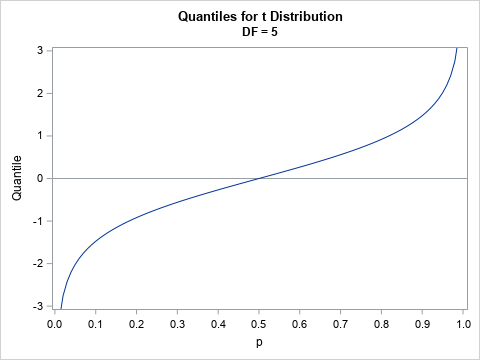Recently, I needed to solve an optimization problem in which the objective function included a term that involved the quantile function (inverse CDF) of the t distribution, which is shown to the right for DF=5 degrees of freedom. I casually remarked to my colleague that the optimizer would have to use finite-difference derivatives because "this objective function does not have an analytical derivative." But even as I said it, I had a nagging thought in my mind. Is that statement true?

I quickly realized that I was wrong. The quantile function of ANY continuous probability distribution has an analytical derivative as long as the density function (PDF) is nonzero at the point of evaluation. Furthermore, the quantile function has an analytical expression for the second derivative if the density function is differential.

This article derives an exact analytical expression for the first and second derivatives of the quantile function of any probability distribution that has a smooth density function. The article demonstrates how to apply the formula to the t distribution and to the standard normal distribution. For the remainder of this article, we assume that the density function is differentiable.

### The derivative of a quantile function

It turns out that I had already solved this problem in a previous article in which I looked at a differential equation that all quantile functions satisfy. In the appendix of that article, I derived a relationship between the first derivative of the quantile function and the PDF function for the same distribution. I also showed that the second derivative of the quantile function can be expressed in terms of the PDF and the derivative of the PDF.

Here are the results from the previous article. Let X be a random variable that has a smooth density function, f. Let w = w(p) be the p_th quantile. Then the first derivative of the quantile function is
$\frac{dw}{dp} = \frac{1}{f(w(p))}$
provided that the denominator is not zero. The second derivative is
$\frac{d^2w}{dp^2} = \frac{-f^\prime(w)}{f(w)} \left( \frac{dw}{dp} \right)^2 = \frac{-f^\prime(w)}{f(w)^3}$.

### Derivatives of the quantile function for the t distribution

Let's confirm the formulas for the quantile function of the t distribution with five degrees of freedom. You can use the PDF function in SAS to evaluate the density function for many common probability distributions, so it is easy to get the first derivative of the quantile function in either the DATA step or in the IML procedure, as follows:

proc iml; reset fuzz=1e-8; DF = 5; /* first derivative of the quantile function w = F^{-1}(p) for p \in (0,1) */ start DTQntl(p) global(DF); w = quantile("t", p, DF); /* w = quantile for p */ fw = pdf("t", w, DF); /* density at w */ return( 1/fw ); finish;   /* print the quantile and derivative at several points */ p = {0.1, 0.5, 0.75}; Quantile = quantile("t", p, DF); /* w = quantile for p */ DQuantile= DTQntl(p); print p Quantile[F=Best6.] DQuantile[F=Best6.];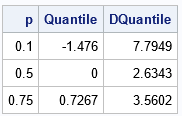This table shows the derivative (third column) for the quantiles of the t distribution. If you look at the graph of the quantile function, these values are the slope of the tangent lines to the curve at the given values of the p variable.

### Second derivatives of the quantile function for the t distribution

With a little more work, you can obtain an analytical expression for the second derivative. It is "more work" because you must use the derivative of the PDF, and that formula is not built-in to most statistical software. However, derivatives are easy (if unwieldy) to compute, so if you can write down the analytical expression for the PDF, you can write down the expression for the derivative.

For example, the following expression is the formula for the PDF of the t distribution with ν degrees of freedom:

$f(x)={\frac {\Gamma ({\frac {\nu +1}{2}})} {{\sqrt {\nu \pi }}\,\Gamma ({\frac {\nu }{2}})}} \left(1+{\frac {x^{2}}{\nu }}\right)^{-(\nu +1)/2}$
Here, $\Gamma$ is the gamma function, which is available in SAS by using the GAMMA function. If you take the derivative of the PDF with respect to x, you obtain the following analytical expression, which you can use to compute the second derivative of the quantile function, as follows:
/* derivative of the PDF for the t distribution */ start Dtpdf(x) global(DF); nu = DF; pi = constant('pi'); A = Gamma( (nu +1)/2 ) / (sqrt(nu*pi) * Gamma(nu/2)); dfdx = A * (-1-nu)/nu # x # (1 + x##2/nu)##(-(nu +1)/2 - 1); return dfdx; finish;   /* second derivativbe of the quantile function for the t distribution */ start D2TQntl(p) global(df); w = quantile("t", p, df); fw = pdf("t", w, df); dfdx = Dtpdf(w); return( -dfdx / fw##3 ); finish;   D2Quantile = D2TQntl(p); print p Quantile[F=Best6.] DQuantile[F=Best6.] D2Quantile[F=Best6.];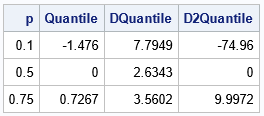### Summary

In summary, this article shows how to compute the first and second derivatives of the quantile function for any probability distribution (with mild assumptions for the density function). The first derivative of the quantile function can be defined in terms of the quantile function and the density function. For the second derivative, you usually need to compute the derivative of the density function. The process is demonstrated by using the t distribution. For the standard normal distribution, you do not need to compute the derivative of the density function; the derivatives depend only on the quantile function and the density function.

The post The derivative of a quantile function appeared first on The DO Loop.

For a linear regression model, a useful but underutilized diagnostic tool is the partial regression leverage plot. Also called the partial regression plot, this plot visualizes the parameter estimates table for the regression. For each effect in the model, you can visualize the following statistics:

• The estimate for each regression coefficient in the model.
• The hypothesis tests β0=0, β1=0, ..., where βi is the regression coefficient for the i_th effect in the model.
• Outliers and high-leverage points.

This article discusses partial regression plots, how to interpret them, and how to create them in SAS. If you are performing a regression that uses k effects and an intercept term, you will get k+1 partial regression plots.

### Example data for partial regression leverage plots

The following SAS DATA step uses Fisher's iris data. To make it easier to discuss the roles of various variables, the DATA step renames the variables. The variable Y is the response, and the explanatory variables are x1, x2, and x3. (Explanatory variables are also called regressors.)

In SAS, you can create a panel of partial regression plots automatically in PROC REG. Make sure to enable ODS GRAPHICS. Then you can use the PARTIAL option on the MODEL statement PROC REG statement to create the panel. To reduce the number of graphs that are produced, the following call to PROC REG uses the PLOTS(ONLY)=(PARTIAL) option to display only the partial regression leverage plots.

data Have; set sashelp.iris(rename=(PetalLength = Y PetalWidth = x1 SepalLength = x2 SepalWidth = x3) where=(Species="Versicolor")); ID = _N_; label Y= x1= x2= x3=; run;   ods graphics on; title "Basic Partial Regression Leverage Plots"; proc reg data=Have plots(only)=(PartialPlot); model Y = x1 x2 x3 / clb partial; ods select ParameterEstimates PartialPlot; quit;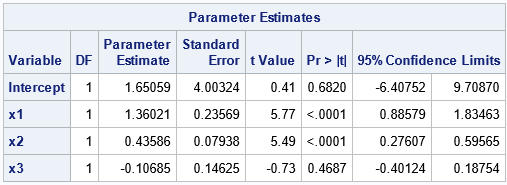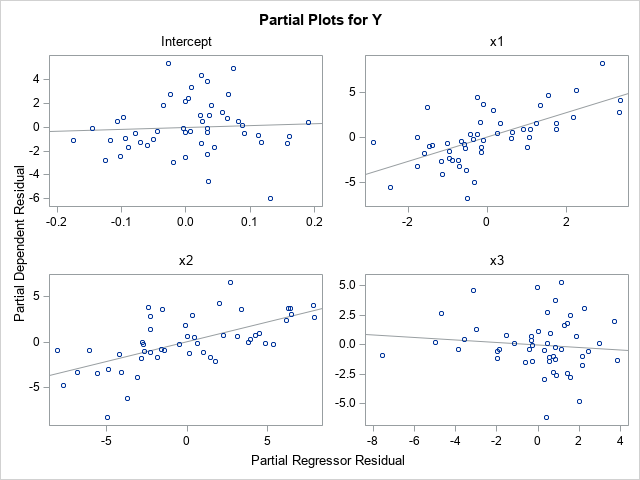Let's call the parameter for the Intercept term the 0th coefficient, the parameter for x1 the 1st coefficient, and so on. Accordingly, we'll call the upper left plot the 0th plot, the upper right plot the 1st plot, and so on.

A partial regression leverage plot is a scatter plot that shows the residuals for a specific regressions model. In the i_th plot (i=0,1,2,3), the vertical axis plots the residuals for the regression model where Y is regressed onto the explanatory variables but omits the i_th variable. The horizontal axis plots the residuals for the regression model where the i_th variable is regressed onto the other explanatory variables. For example:

• The scatter plot with the "Intercept" title is the 0th plot. The vertical axis plots the residual values for the model that regresses Y onto the no-intercept model with regressors x1, x2, and x3. The horizontal axis plots the residual values for the model that regresses the Intercept column in the design matrix onto the regressors x1, x2, and x3. Thus, the regressors in this plot omit the Intercept variable.
• The scatter plot with the "x1" title is the 1st plot. The vertical axis plots the residual values for the model that regresses Y onto the model with regressors Intercept, x2, and x3. The horizontal axis plots the residual values for the model that regresses x1 onto the regressors Intercept, x2, and x3. Thus, the regressors in this plot omit the x1 variable.

These plots are called "partial" regression plots because each plot is based on a regression model that contains only part of the full set of regressors. The i_th plot omits the i_th variable from the set of regressors.

### Interpretation of a partial regression leverage plot

Each partial regression plot includes a regression line. It is this line that makes the plot useful for visualizing the parameter estimates. The line passes through the point (0, 0) in each plot. The slope of the regression line in the i_th plot is the parameter estimate for the i_th regression coefficient (βi) in the full model. If the regression line is close to being horizontal, that is evidence for the null hypothesis βi=0.

To demonstrate these facts, look at the partial regression plot for the Intercept. The partial plot has a regression line that is very flat (almost horizontal). This is because the parameter estimate for the Intercept is 1.65 with a standard error of 4. The 95% confidence interval is [-6.4, 9.7]. Notice that this interval contains 0, which means that the flatness of the regression line in the partial regression plot supports "accepting" (failing to reject) the null hypothesis that the Intercept parameter is 0.

In a similar way, look at the partial regression plot for the x1 variable. The partial plot has a regression line that is not flat. This is because the parameter estimate for x1 is 1.36 with a standard error of 0.24. The 95% confidence interval is [0.89, 1.83], which does not contain 0. Consequently, the steepness of the slope of the regression line in the partial regression plot visualizes the fact that we would reject the null hypothesis that the x1 coefficient is 0.

The partial regression plots for the x2 and x3 variables are similar. The regression line in the x2 plot has a steep slope, so the confidence interval for the x2 parameter does not contain 0. The regression line for the x3 variable has a negative slope because the parameter estimate for x3 is negative. The line is very flat, which indicates that the confidence interval for the x3 parameter contains 0.

John Sall ("Leverage Plots for General Linear Hypotheses", TAS, 1990) showed that you could add a confidence band to the partial regression plot such that if the line segment for Y=0 is not completely inside the band, then you reject the null hypothesis that the regression coefficient is 0.

### Identify outliers by using partial regression leverage plots

Here is a remarkable mathematical fact about the regression line in a partial regression plot: the residual for each observation in the scatter plot is identical to the residual for the same observation in the full regression model! Think about what this means. The full regression model in this example is a set of 50 points in four-dimensional space. The regression surface is a 3-D hyperplane over the {x1, x2, x3} variables. Each observation has a residual, which is obtained by subtracting the predicted value from the observed value of Y. The remarkable fact is that in each partial regression plot, the residuals between the regression lines and the 2-D scatter points are exactly the same as the residuals in the full regression model. Amazing!

One implication of this fact is that you can identify points where the residual value is very small or very large. The small residuals indicate that the model fits these points well; the large residuals are outliers for the model.

Let's identify some outliers for the full model and then locate those observations in each of the partial regression plots. If you run the full regression model and analyze the residual values, you can determine that the observations that have the largest (magnitude of) residuals are ID=15, ID=39, ID=41, and ID=44. Furthermore, the next section will look at high-leverage points, which are ID=2 and ID=3. Unfortunately, the PLOTS=PARTIALPLOT option does not support the LABEL suboption, so we need to output the partial regression data and create the plots manually. The following DATA step adds a label variable to the data and reruns the regression model. The PARTIALDATA option on the MODEL statement creates an ODS table (PartialPlotData) that you can write to a SAS data set by using the ODS OUTPUT statement. That data set contains the coordinates that you need to create all of the partial regression plots manually:

/* add indicator variable for outliers and high-leverage points */ data Have2; set Have; if ID in (2,3) then Special="Leverage"; else if ID in (15,39,41,44) then Special="Outlier "; else Special = "None "; if Special="None" then Label=.; else Label=ID; run;   proc reg data=Have2 plots(only)=PartialPlot; model Y = x1 x2 x3 / clb partial partialdata; ID ID Special Label; ods exclude PartialPlotData; ods output PartialPlotData=PD; quit;

You can use the PD data set to create the partial regression plot. The variables for the horizontal axis have names such as Part_Intercept, Part_x1, and so forth. The variables for the vertical axis have names such as Part_Y_Intercept, Part_Y_x1, and so forth. Therefore, it is easy to write a macro that creates the partial regression plot for any variable.

%macro MakePartialPlot(VarName); title "Partial Leverage Plot for &VarName"; proc sgplot data=PD ; styleattrs datasymbols=(CircleFilled X Plus); refline 0 / axis=y; scatter y=part_Y_&VarName x=part_&VarName / datalabel=Label group=Special; reg y=part_Y_&VarName x=part_&VarName / nomarkers; run; %mend;

The following ODS GRAPHICS statement uses the PUSH and POP options to temporarily set the ATTRPRIORITY option to NONE so that the labeled points appear in different colors and symbols. The program then creates all four partial regression plots and restores the default options:

ods graphics / PUSH AttrPriority=None width=360px height=270px; %MakePartialPlot(Intercept); %MakePartialPlot(x1); %MakePartialPlot(x2); %MakePartialPlot(x3); ods graphics / POP; /* restore ODS GRAPHICS options */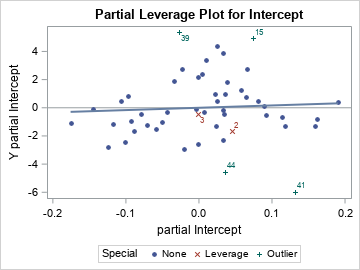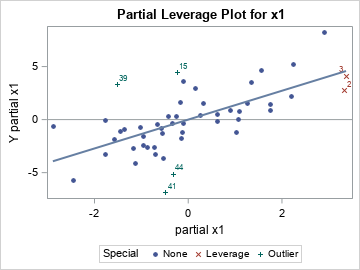The first two plots are shown. The outliers (ID in (15,39,41,44)) will have large residuals in EVERY partial regression plot. In each scatter plot, you can see that the green markers with the "plus" (+) symbol are far from the regression line. Therefore, they are outliers in every scatter plot.

### Identify high-leverage points by using partial regression leverage plots

In a similar way, the partial regression plots enable you to see whether a high-leverage point has extreme values in any partial coordinate. For this example, two high-leverage points are ID=2 and ID=3, and they are displayed as red X-shaped markers.

The previous section showed two partial regression plots. In the partial plot for the Intercept, the two influential observations do not look unusual. However, in the x1 partial plot, you can see that both observations have extreme values (both positive) for the "partial x1" variable.

Let's look at the other two partial regression plots: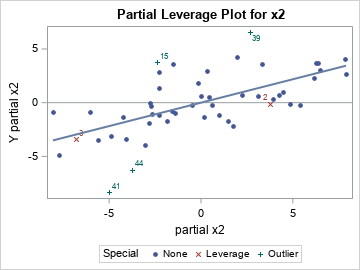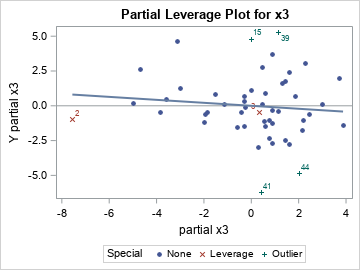The partial regression plot for x2 shows that the "partial x2" coordinate is extreme (very negative) for ID=3. The partial regression plot for x3 shows that the "partial x3" coordinate is extreme (very negative) for ID=2. Remember that these extreme values are not the coordinates of the variables themselves, but of the residuals when you regress each variable onto the other regressors.

It is not easy to explain in a few sentences how the high-leverage points appear in the partial regression plots. I think the details are described in Belsley, Kuh, and Welsch (Regression Diagnostics, 1980), but I cannot check that book right now because I am working from home. But these extreme values are why the Wikipedia article about partial regression plots states, "the influences of individual data values on the estimation of a coefficient are easy to see in this plot."

### Summary

In summary, the partial regression leverage plots provide a way to visualize several important features of a high-dimensional regression problem. The PROC REG documentation includes a brief description of how to create partial regression leverage plots in SAS. As shown in this article, the slope of a partial regression line equals the parameter estimate, and the relative flatness of the line enables you to visualize the null hypothesis βi=0.

This article describes how to interpret the plots to learn more about the regression model. For example, outliers in the full model are also outliers in every partial regression plot. Observations that have small residuals in the full model also have small residuals in every partial regression plot. The high-leverage points will often show up as extreme values in one or more partial regression plots. To examine outliers and high-leverage plots in SAS, you can use the PARTIALDATA option to write the partial regression coordinates to a data set, then add labels for the observations of interest.

Partial regression leverage plots are a useful tool for analyzing the fit of a regression model. They are most useful when the number of observations is not too big. I recommend them when the sample size is 500 or less.

The post Partial leverage plots appeared first on The DO Loop.

The ODS GRAPHICS statement in SAS supports more than 30 options that enable you to configure the attributes of graphs that you create in SAS. Did you know that you can display the current set of graphical options? Furthermore, did you know that you can temporarily set certain options and then restore the options to their previous values? This article shows how to use the SHOW, PUSH, POP, and RESET options on the ODS GRAPHICS statement.

### SHOW the ODS GRAPHICS options

ODS graphics have a default set of characteristics, but you can use the ODS GRAPHICS statement to override the default characteristics for graphs. Probably the most familiar options are the WIDTH= and HEIGHT= options, which set a graph's horizontal and vertical dimensions, respectively. For example, the following ODS GRAPHICS statement resets all options to their default values (RESET) and then sets the dimensions of future graphs. You can use the SHOW option on the ODS GRAPHICS statement to list the default value of several graphical options:

ods graphics / reset width=480px height=360px; ods graphics / SHOW;

The SAS log shows the value of many options. The first few lines are shown below:

ODS Graphics Settings --------------------- Output format: STATIC By line: NOBYLINE Antialias: ON Maximum Loess observations: 5000 Image width: 480px Image height: 360px Maximum stack depth: 1024 Stack depth: 0 ... other options omitted ...

The log reports that the width and height of future graphs are set to 480 pixels and 360 pixels, respectively. Note that the "stack depth" is set to 0, which means that no options have been pushed onto the stack. I will say more about the stack depth in a later section.

To demonstrate that the new options are in effect, the following call to PROC SGPLOT creates a scatter plot of two variables in the SasHelp.Iris data set and uses the GROUP= option to visualize the three different species of flowers in the data:

title "Indicate Groups by Using GROUP= Option"; title2 "HTML Destination Uses AttrPriority=COLOR"; proc sgplot data=sashelp.iris; scatter x=PetalWidth y=SepalWidth/ group=Species; run;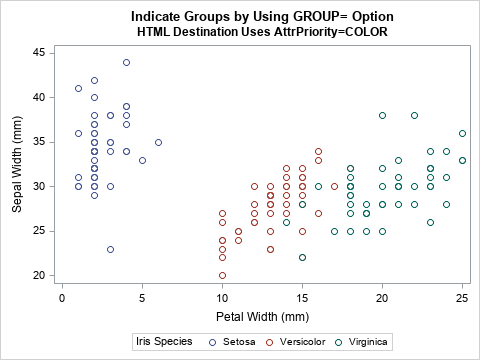This image shows what the graph looks like in the HTML destination, which uses the ATTRPRIORITY=COLOR option by default. When you use the ATTRPRIORITY=COLOR option, groups are visualized by changing the color of markers (or lines). Thus, some markers are blue, others are reddish, and others are green. There is another option (ATTRPRIORITY=NONE), which is used by other ODS destinations, which visualizes groups by changing the color and symbol of markers (and the color and line pattern for lines). For more information about the ATTRPRIORITY= option, see "Getting started with SGPLOT: Style Attributes."

### PUSH new options onto the stack

If you override the default value of an option, it will affect all future graphs until you reset the option or end the SAS session. Often, this is exactly what you want to happen. However, sometimes you want to temporarily override options, create some graphs, and then restore the existing set of options. For example, if you are producing code for others to use (such as writing a SAS macro), it is a good programming practice to not change any options that the user has set. For this situation, you can use the PUSH and POP options on the ODS GRAPHICS statement.

Let's see how the PUSH option works. The following statement temporarily overrides the ATTRPRIORITY= option by pushing the option NONE onto the current stack of options. (If you are not familiar with the stack data structure, read the Wikipedia article about stacks.) The call to PROC SGPLOT then creates a scatter plot. The scatter plot uses both colors and symbols to visualize the species of flowers.

ods graphics / PUSH AttrPriority=None; /* temporarily change option to NONE */   title "Indicate Groups by Using Symbols"; title2 "Use AttrPriority=NONE"; proc sgplot data=sashelp.iris; scatter x=PetalWidth y=SepalWidth/ group=Species; run;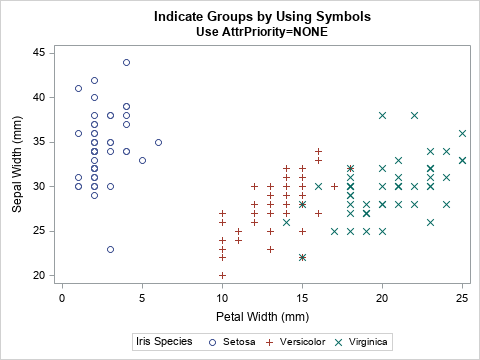You can confirm that the options have changed by using the SHOW option to see the new values of the ODS GRAPHICS options:

ods graphics / SHOW;
ODS Graphics Settings --------------------- Output format: STATIC By line: NOBYLINE Antialias: ON Maximum Loess observations: 5000 Image width: 480px Image height: 360px Attribute priority: NONE Maximum stack depth: 1024 Stack depth: 1 ... other options omitted ...

The SAS log confirms that the ATTRPRIORITY= option is set to NONE. In addition, the "stack depth" value is now 1, which means that you have pushed options onto the stack. Additional ODS GRAPHICS statements will affect the state of this stack, but they are "temporary" in the sense that you can use the POP option to restore the previous options, as shown in the next section.

### POP the stack to restore old options

If you use the PUSH option to create a new set of options, you can use the POP option to restore the previous options. For example, the following statement pops the stack and displays the current options to the SAS log:

ods graphics / POP; /* restore previous options */ ods graphics / SHOW;
ODS Graphics Settings --------------------- Output format: STATIC By line: NOBYLINE Antialias: ON Maximum Loess observations: 5000 Image width: 480px Image height: 360px Maximum stack depth: 1024 Stack depth: 0 ... other options omitted ...

The log shows that the ATTRPRIORITY= option is no longer set, which means that each destination will use its default behavior. In addition, the stack depth is back to 0, which means that the options that are displayed are the "base" options at the bottom of the stack. Notice that the image width and height are not the default values because those options were set before the first PUSH option was set.

### RESET all or some options

Even though the width and height options were set before the first PUSH operation, you can still restore the width and height to their default values. The RESET= option can reset individual options, as follows:

ods graphics / reset=width reset=height; /* restore width and height to default values */

You can restore all options to their default values by using the RESET=ALL option. Alternatively, you can use the RESET option without an equal sign.

/* restore all options to default values */ ods graphics / reset=all; /* same as ODS GRAPHICS / RESET; */

### Summary

The ODS GRAPHICS statement enables you to set options that affect the characteristics of future graphs. If you want to temporarily change the options, you can use the PUSH option to add options to the stack. All future graphs will use those options. You can restore the previous set of options by using the POP option to pop the stack. If you are writing code for others to use (for example, a SAS macro), it is good programming practice to push your options onto a stack and then pop the options after your program completes. In this way, you will not overwrite options that the use of your program has explicitly set.

If you set an option but later want to restore it to its default value, you can use the RESET= option.

The post PUSH, POP, and reset options for ODS graphics appeared first on The DO Loop.

A palindrome is a sequence of letters that is the same when read forward and backward. In brief, if you reverse the sequence of letters, the word is unchanged. For example, 'mom' and 'racecar' are palindromes. You can extend the definition to phrases by removing all spaces and punctuation marks from the phrase and asking whether the remaining letters are a palindrome. For example, 'borrow or rob?' is a palindrome because the sequence of letters 'borroworrob' is the same when read forward and backward.

Recently, I saw a new kind of palindrome: The rotational palindrome. You take a word (written in lowercase) and rotate it 180 degrees so that the letters are upside down and reversed. You then ask whether the pattern of letters matches the original word. If so, you have found a rotational palindrome!

This article shows how to detect palindromes, palindrome phrases, and rotational palindromes in SAS. Along the way, we learn about a few useful string-comparison functions, such as the UPCASE, STRIP, COMPRESS, REVERSE, and TRANSLATE functions.

### Detect a palindrome word in SAS

To introduce the topic of palindromes, let's first consider only words. If you want to determine whether a word is a palindrome, do the following:

1. Use the UPCASE function to convert all letters to uppercase.
2. Use the REVERSE function to obtain the reversed sequence of letters.
3. Use the STRIP function to remove any leading or trailing blanks. Compare the original and the reversed sequence of letters. If the two sequences are the same, the word is a palindrome.

The following SAS DATA step implements this algorithm on a series of words.

data Words; length source $120; input source; datalines; solos Hannah Dad madaM racecar SAS STATs programmer ; /* detect palindrome words */ data Palindrome; set Words; length str reverse$120; str = upcase(source); reverse = reverse(str); isPalindrome = (strip(str) = strip(reverse)); /* 0/1 indicator variable */ run;   proc print noobs; run;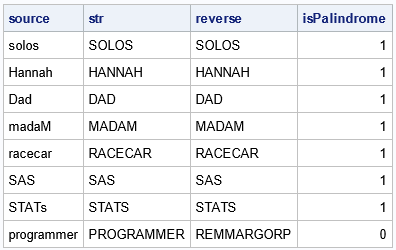The data set contains an indicator variable (isPalindrome), which has the value 1 for palindrome words and the value 0 if the word is not a palindrome. Words like 'solos', 'dad', and 'SAS' are palindromes. The word 'programmer' is not.

### Detect a palindrome phrase in SAS

The same logic works for a palindromic phrase but requires an extra step. For a phrase, you must first remove blanks and punctuation from the string, then apply the preceding logic to the remaining sequence of letters. You can use the COMPRESS function in SAS to remove blanks and punctuation. Often, programmers call the COMPRESS function with two arguments: the input string and a set of characters to remove from the string. However, the optional third argument provides a way to remove common sets of characters from the input string. For example, to remove all punctuation characters, you can specify 'p' as part of the third argument. To remove all whitespace, you can specify the 's' modifier. You can specify 'ps' to combine those two modifiers, as done below:

data Sentences; length source $120; infile datalines truncover; input source$ 1-120; /* use DATALINES4 if a string includes a semicolon */ datalines; Madam, I'm Adam. Step on no pets. No lemon, no melon. To be or not to be. My gym, sir, is my gym. Was it a cat I saw? A man, a plan, a canal: Panama! racecar SAS programmer ;   /* detect palindrome phrases (also works for words) */ data Palindrome; set sentences; length str reverse $120; str = compress(upcase(source), , 'ps'); /* remove punctuation and whitespace */ reverse = reverse(str); palindrome = (str = strip(reverse)); /* no blanks in str */ drop reverse; run; proc print noobs; run;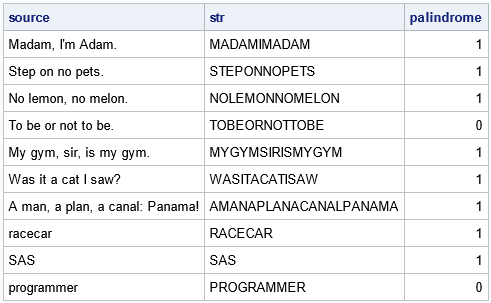This program handles words as well as phrases, so it can replace the program in the previous section. As a boy, I encountered the palindromic phrase "A man, a plan, a canal: Panama!" in a book of word games; it has always amused me! ### Generalizations of palindromes The traditional palindrome is a specific example of a more general mathematical problem. Mathematically, a transformation is a function that maps a set into itself. A fixed point is an element in the domain of a transformation that is mapped to itself. If R is the transformation on a set of letters that reverses the letters, then a palindrome is a fixed point for the R transformation. With this generalization, you can play all sorts of mathematical games. You can search for the closest palindrome to a given word. You can search for "near palindromes." For example, 'bevel' is a near palindrome because you can replace the 'b' with an 'l' to obtain the palindrome 'level'. Alternatively, you can change the transformation and find fixed points of the new transformation. This is the idea behind a rotational palindrome. Certain lowercase letters look like a letter when you rotate them by 180 degrees. I use the phrase rotationally similar to refer to a pair of letters like 'b' and 'q' that are 180-degree rotations of each other. Some letters (such as 'o' and 's') are rotationally similar to themselves. Given a set of letters, define a transformation in two steps: first map certain letters to their rotationally similar image. Next, apply the REVERSE transformation. You then search for fixed points of the composite mapping. Each of the following 13 lowercase letters is rotationally similar to another lowercase letter. Five of them ('l', 'o', 's', 'x', and 'z') are self-similar: b -> q d -> p l -> l m -> w n -> u o -> o p -> d q -> b s -> s u -> n w -> m x -> x z -> z To completely define the transformation, you should also define how it acts on the remaining letters ('a', 'c', 'e', etc.). Words that contain these letters are never rotational palindromes because rotating these letters by 180 degrees does not result in a valid lowercase letter.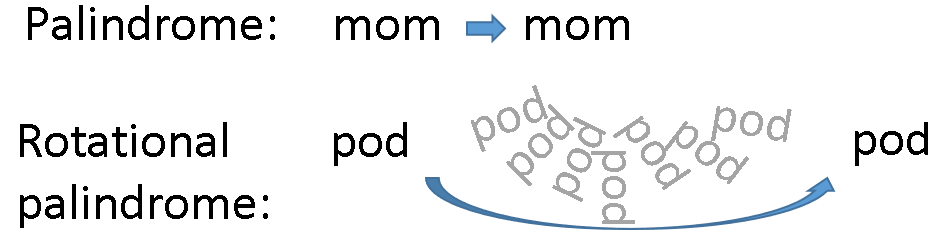For example, 'mom' is not a rotational palindrome because if you rotate it 180 degrees you get 'wow'. But 'pod' is a rotational palindrome because the letter 'p' is the 180-degree rotation of 'd' (and vice versa) and the letter 'o' is rotationally symmetric. The word 'mow' is a rotational palindrome, but 'maw' is not because the letter 'a' is not rotationally similar to any other letter. ### Rotational palindromes in SAS You can use the TRANSLATE function in SAS to define the rotational transformation. The TRANSLATE function takes three arguments: the input string and two replacement strings, TO and FROM. The FROM string is the set of letters that you want to replace, and the TO string is the set of letters that you want to use for the replacement. I think it is clearest to define a string that has 26 letters so that you can see how every letter in a word is transformed. In the following program, the letters that do not have rotational cousins are assigned to a period (.). Assuming that you remove all punctuation from the input string, this choice will ensure that the program will correctly identify rotational palindromes. You can use a mathematical trick to check for a rotational palindrome. A rotation by 180 degrees is mathematically equivalent to a horizontal reflection followed by a vertical reflection. Therefore, you can program a 180-degree rotation by first substituting the rotationally similar letter and then reversing the string, as follows: data Words2; length source$120; infile datalines truncover; input source $1-120; datalines; solos mow maw pod dad mop dow madam SAS ; /* detect rotational palindromes */ data Rotate; set Words2; length str flip flipReverse$120; FROM='abcdefghijklmnopqrstuvwxyz0123456789'; /* old values (including digits) */ TO = '.q.p.......lwuodb.s.n.mx.z01....9.86'; /* map to new values */ str = compress(lowcase(source), , 'ps'); /* remove blanks and punctuation */ flip = translate(str, TO, FROM); /* map to rotationally similar letters */ flipReverse = strip(reverse(flip)); /* do the usual palindrome check */ isRotPalindrome = (str = flipReverse); drop TO FROM; run;   proc print noobs; run;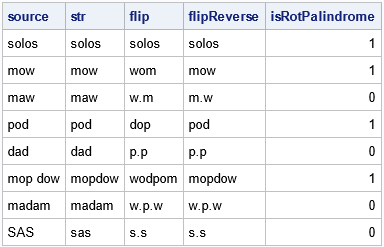The program removes punctuation and spaces, translates each letter to its rotationally similar image, then determines whether the reversed string is equal to the original string. The program verifies that 'mow' and 'pod' are rotational palindromes. In addition, notice that the word 'solos' is a very special word. It is both a traditional palindrome and a rotational palindrome! A palindrome that contains only self-similar letters is also a rotational palindrome.

### Summary

A traditional palindrome is a string of letters that are the same in forward and reversed order. This article shows how to use built-in string functions in SAS to detect palindromes. Some useful functions include UPCASE, STRIP, COMPRESS, and REVERSE. In addition, you can generalize the notion of a palindrome. A generalized palindrome is a fixed point for a transformation of the letters in a string. I show how to define a rotational palindrome by mapping certain letters to their rotationally similar images, then checking whether the reversal of the resulting string is equal to the original string.

Feel free to share any thoughts you might have regarding palindromes. Do you think rotational palindromes are interesting? Can you find any words that are longer than five letters and are rotational palindromes? Can you think of other interesting transformations of strings? What are the fixed points (palindromes) for those transformations?

The post Detect palindromes and rotational palindromes in SAS appeared first on The DO Loop.

M estimation is a robust regression technique that assigns a weight to each observation based on the magnitude of the residual for that observation. Large residuals are downweighted (assigned weights less than 1) whereas observations with small residuals are given weights close to 1. By iterating the reweighting and fitting steps, the method converges to a regression that de-emphasizes observations whose residuals are much bigger than the others. This iterative process is also known called iteratively reweighted least squares.

A previous article visualizes 10 different "weighting functions" that are supported by the ROBUSTREG procedure in SAS. That article mentions that the results of the M estimation process depend on the choice of the weighting function. This article runs three robust regression models on the same data. The only difference between the three models is the choice of a weighting function. By comparing the regression estimates for each model, you can understand how the choice of a weighting function affects the final model.

### Data for robust regression

The book Applied Regression Analysis and Generalized Linear Models (3rd Ed, J. Fox, 2016) analyzes Duncan's occupational prestige data (O. D. Duncan, 1961), which relates prestige to the education and income of men in 45 occupations in the US in 1950. The following three variables are in the data set:

• Income (Explanatory): Percent of males in the occupation who earn $3,500 or more. • Education (Explanatory): Percent of males in the occupation who are high-school graduates. • Prestige (Response): Percent of raters who rated the prestige of the occupation as excellent or good. The following scatter plot shows the distributions of the Income and Education variables. The color of the marker reflects the Prestige variable. Certain occupations are labeled: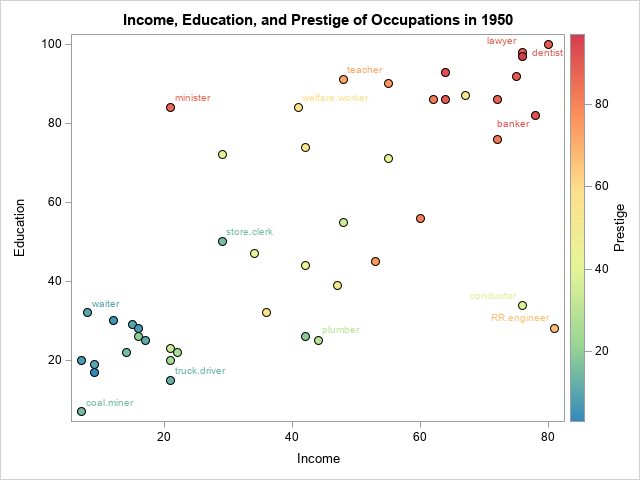The markers are roughly divided into four regions: • The cluster of markers in the lower-left corner of the graph are unskilled laborers such as coal miners, truck drivers, and waiters. The men in these occupations have little education and earn little income. • Markers in the lower-right corner represent are skilled laborers such as plumbers and railroad conductors who make considerable income despite having little education. • Markers in the upper-left corner represent occupations such as ministers and welfare workers who have considerable education but low-paying jobs. • Markers in the upper-right corner represent occupations such as doctors, lawyers, and bankers who have a lot of education and high income. In general, the prestige of an occupation increases from lower-left to upper-right. However, there are some exceptions. A minister has more prestige than might be expected from his income. Reporters and railroad conductors have less prestige than you might expect from their income. A robust regression model that uses M estimation will assign smaller weights to observations that do not match the major trends in the data. Observations that have very small weights are classified as outliers. The following SAS DATA step creates Duncan's prestige data: /* Duncan's Occupational Prestige Data from John Fox (2016), Applied Regression Analysis and Generalized Linear Models, Third Edition https://socialsciences.mcmaster.ca/jfox/Books/Applied-Regression-3E/datasets/Duncan.txt Original source: Duncan, O. D. (1961) A socioeconomic index for all occupations. In Reiss, A. J., Jr. (Ed.) Occupations and Social Status. Free Press [Table VI-1]. Type: prof=Professional, wc=White collar, bc=Blue collar */ data Duncan; length Occupation$20 Type $4; input Occupation Type Income Education Prestige; ObsNum + 1; datalines; accountant prof 62 86 82 pilot prof 72 76 83 architect prof 75 92 90 author prof 55 90 76 chemist prof 64 86 90 minister prof 21 84 87 professor prof 64 93 93 dentist prof 80 100 90 reporter wc 67 87 52 engineer prof 72 86 88 undertaker prof 42 74 57 lawyer prof 76 98 89 physician prof 76 97 97 welfare.worker prof 41 84 59 teacher prof 48 91 73 conductor wc 76 34 38 contractor prof 53 45 76 factory.owner prof 60 56 81 store.manager prof 42 44 45 banker prof 78 82 92 bookkeeper wc 29 72 39 mail.carrier wc 48 55 34 insurance.agent wc 55 71 41 store.clerk wc 29 50 16 carpenter bc 21 23 33 electrician bc 47 39 53 RR.engineer bc 81 28 67 machinist bc 36 32 57 auto.repairman bc 22 22 26 plumber bc 44 25 29 gas.stn.attendant bc 15 29 10 coal.miner bc 7 7 15 streetcar.motorman bc 42 26 19 taxi.driver bc 9 19 10 truck.driver bc 21 15 13 machine.operator bc 21 20 24 barber bc 16 26 20 bartender bc 16 28 7 shoe.shiner bc 9 17 3 cook bc 14 22 16 soda.clerk bc 12 30 6 watchman bc 17 25 11 janitor bc 7 20 8 policeman bc 34 47 41 waiter bc 8 32 10 ; ### Analysis 1: The bisquare weighting function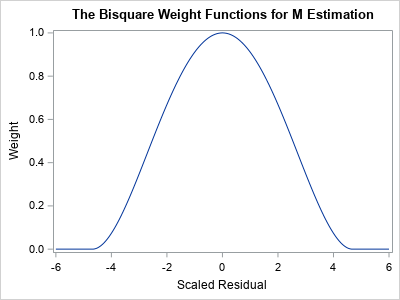The following call to PROC ROBUSTREG shows how to run a robust regression analysis of the Duncan prestige data. The METHOD=M option tells the procedure to use M estimation. The WEIGHTFUNCTION= suboption specifies the weight function that will assign weights to observations based on the size of the residuals. The default weight function is the bisquare function, but the following statements specify the weight function explicitly. A graph of the bisquare weighting function is shown to the right. Observations that have a small residual receive a weight near 1; outliers receive a weight close to 0. title "Robust Regression Analysis for WEIGHTFUNCTION=Bisquare"; proc robustreg data=Duncan plots=RDPlot method=M(WEIGHTFUNCTION=Bisquare); model Prestige = Income Education; ID Occupation; output out=RROut SResidual=StdResid Weight=Weight Leverage=Lev Outlier=Outlier; ods select ParameterEstimates RDPlot; run;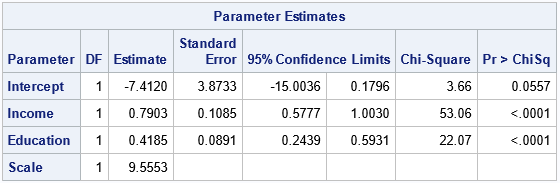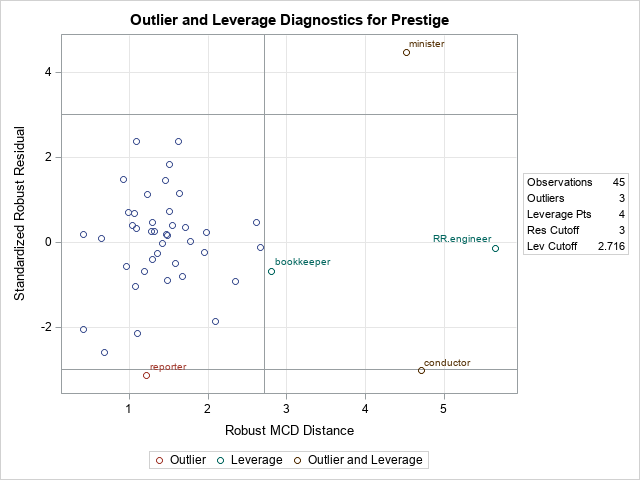The parameter estimates are shown. The bisquare-weighted regression model is Prestige = -7.41 + 0.79*Income + 0.42*Education. The intercept term is not significant at the 0.05 significance level, so you could rerun the analysis to use a no-intercept model. We will not do that here. The plot is called the residual-distance plot (RD plot). You can use an RD plot to identify high-leverage points and outliers for the model. This is a robust version of the residual-leverage plot that is used for ordinary least-squares regression. • The horizontal direction is a robust measure of the leverage. Think of it as a robust distance from the coordinates of each observation to a robust estimate of the center of the explanatory variables. Observations that have a large distance are high-leverage points. M estimation is not robust to the presence of high-leverage points, so SAS will display a WARNING in the log that states "The data set contains one or more high-leverage points, for which M estimation is not robust." You can see from this plot (or from the scatter plot in the previous section) that bookkeepers, ministers, conductors, and railroad engineers are somewhat far from the center of the point cloud in the bivariate space of the explanatory variables (Income and Education). Therefore, they are high-leverage points. • The vertical direction shows the size of a standardized residual for the robust regression model. Reference lines are drawn at ±3. If an observation has a residual that exceeds these values, it is considered an outlier, meaning that the value of Prestige in the data is much higher or lower than the value predicted by the model. You can see from this plot that ministers, reporters, and conductors are outliers for this model. The graph of the bisquare weighting function enables you to mentally estimate the weights for each observation. But an easier way to visualize the weights is to use the WEIGHT= option on the OUTPUT statement to output the weights to a SAS data set. You can then plot the weight for each observation and label the markers that have small weights, as follows: data RROut2; set RROut; length Label$20; if Weight < 0.8 then Label = Occupation; else Label = ""; run;   title "Weights for WEIGHTFUNCTION=Bisquare"; proc sgplot data=RROut2; scatter x=ObsNum y=Weight / datalabel=Label; run;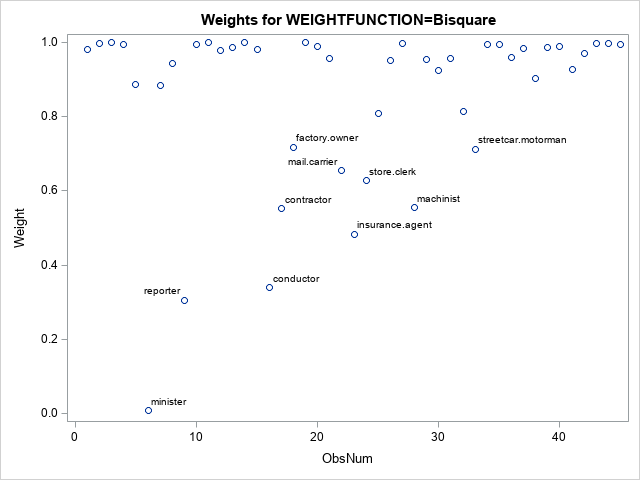The scatter plot (click to enlarge) shows the weights for all observations. A few that have small weights (including the outliers) are labeled. You can see that the minister has a weight close to Weight=0 whereas reporters and conductors have weights close to Weight=0.3. These weights are used in a weighted regression, which is the final regression model.

If you prefer a tabular output, you can use the LEVERAGE= and OUTLIER= options on the OUTPUT statement to output indicator variables that identify each observation as a high-leverage point, an outlier, or both. The following call to PROC PRINT shows how to display only the outliers and high-leverage points:

proc print data=RROut; where Lev=1 | Outlier=1; var Occupation Weight Prestige Income Education Lev Outlier; run;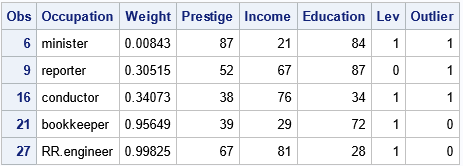The output shows that four observations are high-leverage points and three are outliers. (The minister and reporter occupations are classified as both.) This outlier classification depends on the weighting function that you use during the M estimation procedure.

### Create a SAS macro to repeat the analysis

You can repeat the previous analysis for other weighting functions to see how the choice of a weighting function affects the final regression fit. It makes sense to wrap up the main functionality into a SAS macro that takes the name of the weighting function as an argument, as follows:

%macro RReg( WeightFunc ); title "Robust Regression Analysis for WEIGHTFUNCTION=&WeightFunc"; proc robustreg data=Duncan plots=RDPlot method=M(WEIGHTFUNCTION=&WeightFunc); model Prestige = Income Education; ID Occupation; output out=RROut SResidual=StdResid Weight=Weight; ods select ParameterEstimates RDPlot; run;   data RROut2; set RROut; length Label $20; if Weight < 0.8 then Label = Occupation; else Label = ""; run; title "Weights for WEIGHTFUNCTION=&WeightFunc"; proc sgplot data=RROut2; scatter x=ObsNum y=Weight / datalabel=Label; run; %mend; The next sections call the macro for the Huber and Talworth weighting functions, but you can use it for any of the 10 weighting functions that PROC ROBUSTREG supports. ### Analysis 2: The Huber weighting function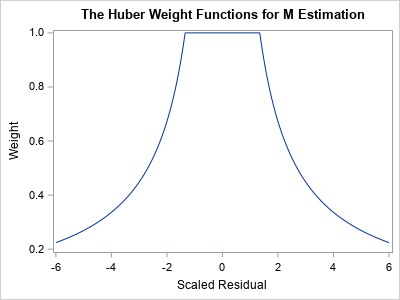A graph of the Huber weight function is shown to the right. Notice that the any observation whose standardized residual is less than 1.345 receives a weight of 1. Observations that have larger residuals are downweighted by using a weight that is inversely proportional to the magnitude of the residual. The following statement re-runs the M estimation analysis, but uses the Huber weighting function: %RReg( Huber ); The parameter estimates are not shown, but the Huber-weighted regression model is Prestige = -7.1107 + 0.70*Income + 0.49*Education. Notice that the estimates are different from the bisquare analysis because the Huber and bisquare functions result in different weights. For this model, reporters and ministers are outliers. Many observations receive full weight (Weight=1) in the analysis. A handful of observations are downweighted, as shown in the following scatter plot (click to enlarge):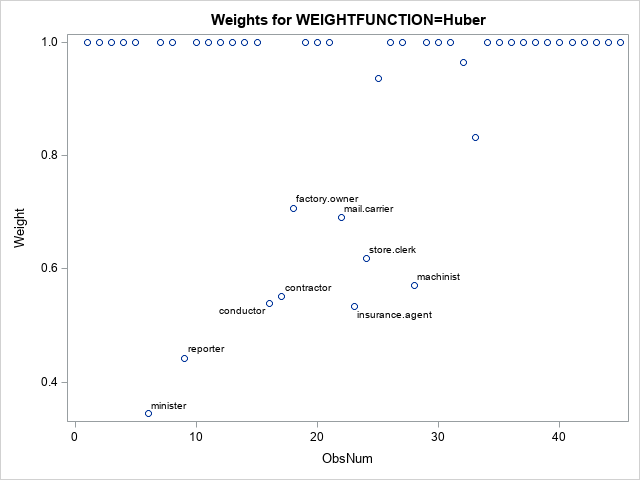### Analysis 3: The Talworth weighting function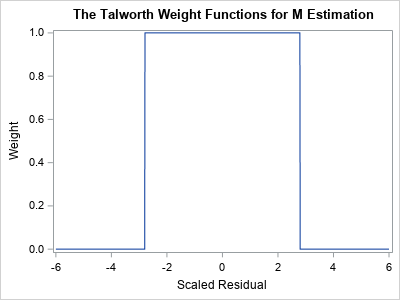A graph of the Talworth weight function is shown to the right. Notice that any observation whose standardized residual is less than 2.795 receives a weight of 1. All other observations are excluded from the analysis by assigning them a weight of 0. The following statement re-runs the M estimation analysis, but uses the Talworth weighting function: %RReg( Talworth ); The parameter estimates are not shown, but the Talworth-weighted regression model is Prestige = -7.45 + 0.74*Income + 0.46*Education. Again, the estimates are different from the estimates that used the other weighting functions. For this analysis, the reporters and ministers are outliers. All other observations receive Weight=1. The weights are shown in the following scatter plot (click to enlarge):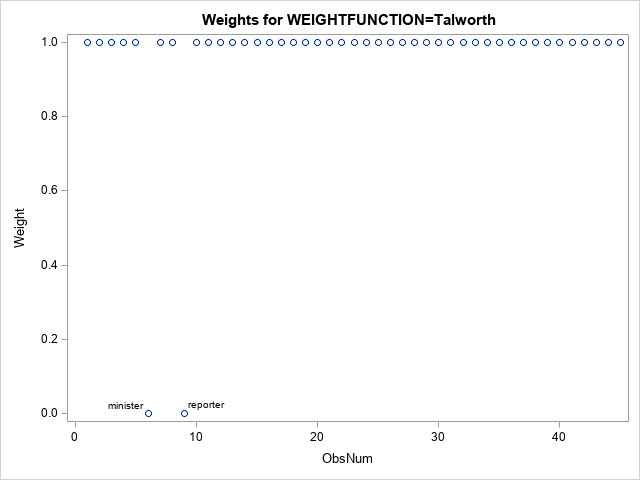### Summary Many statistical procedures depend on parameters, which affect the results of the procedure. Users of SAS software often accept the default methods, but it is important to recognize that an analysis can and will change if you use different parameters. For the ROBUSTREG procedure, the default method is M estimation, and the default weighting function is the bisquare weighting function. This article demonstrates that the fitted regression model will change if you use a different weighting function. Different weighting functions can affect which observations are classified as outliers. Each function assigns different weights to observations that have large residual values. The post The effect of weight functions in a robust regression method appeared first on The DO Loop. M estimation is a robust regression technique that assigns a weight to each observation based on the magnitude of the residual for that observation. Large residuals are downweighted (assigned weights less than 1) whereas observations with small residuals are given weights close to 1. By iterating the reweighting and fitting steps, the method converges to a regression that de-emphasizes observations whose residuals are much bigger than the others. This iterative process is also known called iteratively reweighted least squares. A previous article visualizes 10 different "weighting functions" that are supported by the ROBUSTREG procedure in SAS. That article mentions that the results of the M estimation process depend on the choice of the weighting function. This article runs three robust regression models on the same data. The only difference between the three models is the choice of a weighting function. By comparing the regression estimates for each model, you can understand how the choice of a weighting function affects the final model. ### Data for robust regression The book Applied Regression Analysis and Generalized Linear Models (3rd Ed, J. Fox, 2016) analyzes Duncan's occupational prestige data (O. D. Duncan, 1961), which relates prestige to the education and income of men in 45 occupations in the US in 1950. The following three variables are in the data set: • Income (Explanatory): Percent of males in the occupation who earn$3,500 or more.
• Education (Explanatory): Percent of males in the occupation who are high-school graduates.
• Prestige (Response): Percent of raters who rated the prestige of the occupation as excellent or good.

The following scatter plot shows the distributions of the Income and Education variables. The color of the marker reflects the Prestige variable. Certain occupations are labeled:The markers are roughly divided into four regions:

• The cluster of markers in the lower-left corner of the graph are unskilled laborers such as coal miners, truck drivers, and waiters. The men in these occupations have little education and earn little income.
• Markers in the lower-right corner represent are skilled laborers such as plumbers and railroad conductors who make considerable income despite having little education.
• Markers in the upper-left corner represent occupations such as ministers and welfare workers who have considerable education but low-paying jobs.
• Markers in the upper-right corner represent occupations such as doctors, lawyers, and bankers who have a lot of education and high income.

In general, the prestige of an occupation increases from lower-left to upper-right. However, there are some exceptions. A minister has more prestige than might be expected from his income. Reporters and railroad conductors have less prestige than you might expect from their income. A robust regression model that uses M estimation will assign smaller weights to observations that do not match the major trends in the data. Observations that have very small weights are classified as outliers.

The following SAS DATA step creates Duncan's prestige data:

/* Duncan's Occupational Prestige Data from John Fox (2016), Applied Regression Analysis and Generalized Linear Models, Third Edition https://socialsciences.mcmaster.ca/jfox/Books/Applied-Regression-3E/datasets/Duncan.txt Original source: Duncan, O. D. (1961) A socioeconomic index for all occupations. In Reiss, A. J., Jr. (Ed.) Occupations and Social Status. Free Press [Table VI-1].   Type: prof=Professional, wc=White collar, bc=Blue collar */ data Duncan; length Occupation $20 Type$4; input Occupation Type Income Education Prestige; ObsNum + 1; datalines; accountant prof 62 86 82 pilot prof 72 76 83 architect prof 75 92 90 author prof 55 90 76 chemist prof 64 86 90 minister prof 21 84 87 professor prof 64 93 93 dentist prof 80 100 90 reporter wc 67 87 52 engineer prof 72 86 88 undertaker prof 42 74 57 lawyer prof 76 98 89 physician prof 76 97 97 welfare.worker prof 41 84 59 teacher prof 48 91 73 conductor wc 76 34 38 contractor prof 53 45 76 factory.owner prof 60 56 81 store.manager prof 42 44 45 banker prof 78 82 92 bookkeeper wc 29 72 39 mail.carrier wc 48 55 34 insurance.agent wc 55 71 41 store.clerk wc 29 50 16 carpenter bc 21 23 33 electrician bc 47 39 53 RR.engineer bc 81 28 67 machinist bc 36 32 57 auto.repairman bc 22 22 26 plumber bc 44 25 29 gas.stn.attendant bc 15 29 10 coal.miner bc 7 7 15 streetcar.motorman bc 42 26 19 taxi.driver bc 9 19 10 truck.driver bc 21 15 13 machine.operator bc 21 20 24 barber bc 16 26 20 bartender bc 16 28 7 shoe.shiner bc 9 17 3 cook bc 14 22 16 soda.clerk bc 12 30 6 watchman bc 17 25 11 janitor bc 7 20 8 policeman bc 34 47 41 waiter bc 8 32 10 ;

### Analysis 1: The bisquare weighting functionThe following call to PROC ROBUSTREG shows how to run a robust regression analysis of the Duncan prestige data. The METHOD=M option tells the procedure to use M estimation. The WEIGHTFUNCTION= suboption specifies the weight function that will assign weights to observations based on the size of the residuals.

The default weight function is the bisquare function, but the following statements specify the weight function explicitly. A graph of the bisquare weighting function is shown to the right. Observations that have a small residual receive a weight near 1; outliers receive a weight close to 0.

title "Robust Regression Analysis for WEIGHTFUNCTION=Bisquare"; proc robustreg data=Duncan plots=RDPlot method=M(WEIGHTFUNCTION=Bisquare); model Prestige = Income Education; ID Occupation; output out=RROut SResidual=StdResid Weight=Weight Leverage=Lev Outlier=Outlier; ods select ParameterEstimates RDPlot; run;The parameter estimates are shown. The bisquare-weighted regression model is
Prestige = -7.41 + 0.79*Income + 0.42*Education.
The intercept term is not significant at the 0.05 significance level, so you could rerun the analysis to use a no-intercept model. We will not do that here.

The plot is called the residual-distance plot (RD plot). You can use an RD plot to identify high-leverage points and outliers for the model. This is a robust version of the residual-leverage plot that is used for ordinary least-squares regression.

• The horizontal direction is a robust measure of the leverage. Think of it as a robust distance from the coordinates of each observation to a robust estimate of the center of the explanatory variables. Observations that have a large distance are high-leverage points. M estimation is not robust to the presence of high-leverage points, so SAS will display a WARNING in the log that states "The data set contains one or more high-leverage points, for which M estimation is not robust." You can see from this plot (or from the scatter plot in the previous section) that bookkeepers, ministers, conductors, and railroad engineers are somewhat far from the center of the point cloud in the bivariate space of the explanatory variables (Income and Education). Therefore, they are high-leverage points.
• The vertical direction shows the size of a standardized residual for the robust regression model. Reference lines are drawn at ±3. If an observation has a residual that exceeds these values, it is considered an outlier, meaning that the value of Prestige in the data is much higher or lower than the value predicted by the model. You can see from this plot that ministers, reporters, and conductors are outliers for this model.

The graph of the bisquare weighting function enables you to mentally estimate the weights for each observation. But an easier way to visualize the weights is to use the WEIGHT= option on the OUTPUT statement to output the weights to a SAS data set. You can then plot the weight for each observation and label the markers that have small weights, as follows:

data RROut2; set RROut; length Label $20; if Weight < 0.8 then Label = Occupation; else Label = ""; run; title "Weights for WEIGHTFUNCTION=Bisquare"; proc sgplot data=RROut2; scatter x=ObsNum y=Weight / datalabel=Label; run;The scatter plot (click to enlarge) shows the weights for all observations. A few that have small weights (including the outliers) are labeled. You can see that the minister has a weight close to Weight=0 whereas reporters and conductors have weights close to Weight=0.3. These weights are used in a weighted regression, which is the final regression model. If you prefer a tabular output, you can use the LEVERAGE= and OUTLIER= options on the OUTPUT statement to output indicator variables that identify each observation as a high-leverage point, an outlier, or both. The following call to PROC PRINT shows how to display only the outliers and high-leverage points: proc print data=RROut; where Lev=1 | Outlier=1; var Occupation Weight Prestige Income Education Lev Outlier; run;The output shows that four observations are high-leverage points and three are outliers. (The minister and reporter occupations are classified as both.) This outlier classification depends on the weighting function that you use during the M estimation procedure. ### Create a SAS macro to repeat the analysis You can repeat the previous analysis for other weighting functions to see how the choice of a weighting function affects the final regression fit. It makes sense to wrap up the main functionality into a SAS macro that takes the name of the weighting function as an argument, as follows: %macro RReg( WeightFunc ); title "Robust Regression Analysis for WEIGHTFUNCTION=&WeightFunc"; proc robustreg data=Duncan plots=RDPlot method=M(WEIGHTFUNCTION=&WeightFunc); model Prestige = Income Education; ID Occupation; output out=RROut SResidual=StdResid Weight=Weight; ods select ParameterEstimates RDPlot; run; data RROut2; set RROut; length Label$20; if Weight < 0.8 then Label = Occupation; else Label = ""; run;   title "Weights for WEIGHTFUNCTION=&WeightFunc"; proc sgplot data=RROut2; scatter x=ObsNum y=Weight / datalabel=Label; run; %mend;

The next sections call the macro for the Huber and Talworth weighting functions, but you can use it for any of the 10 weighting functions that PROC ROBUSTREG supports.

### Analysis 2: The Huber weighting functionA graph of the Huber weight function is shown to the right. Notice that the any observation whose standardized residual is less than 1.345 receives a weight of 1. Observations that have larger residuals are downweighted by using a weight that is inversely proportional to the magnitude of the residual.

The following statement re-runs the M estimation analysis, but uses the Huber weighting function:

%RReg( Huber );

The parameter estimates are not shown, but the Huber-weighted regression model is
Prestige = -7.1107 + 0.70*Income + 0.49*Education.
Notice that the estimates are different from the bisquare analysis because the Huber and bisquare functions result in different weights.

For this model, reporters and ministers are outliers. Many observations receive full weight (Weight=1) in the analysis. A handful of observations are downweighted, as shown in the following scatter plot (click to enlarge):### Analysis 3: The Talworth weighting functionA graph of the Talworth weight function is shown to the right. Notice that any observation whose standardized residual is less than 2.795 receives a weight of 1. All other observations are excluded from the analysis by assigning them a weight of 0.

The following statement re-runs the M estimation analysis, but uses the Talworth weighting function:

%RReg( Talworth );

The parameter estimates are not shown, but the Talworth-weighted regression model is
Prestige = -7.45 + 0.74*Income + 0.46*Education.
Again, the estimates are different from the estimates that used the other weighting functions.

For this analysis, the reporters and ministers are outliers. All other observations receive Weight=1. The weights are shown in the following scatter plot (click to enlarge):### Summary

Many statistical procedures depend on parameters, which affect the results of the procedure. Users of SAS software often accept the default methods, but it is important to recognize that an analysis can and will change if you use different parameters. For the ROBUSTREG procedure, the default method is M estimation, and the default weighting function is the bisquare weighting function. This article demonstrates that the fitted regression model will change if you use a different weighting function. Different weighting functions can affect which observations are classified as outliers. Each function assigns different weights to observations that have large residual values.

The post The effect of weight functions in a robust regression method appeared first on The DO Loop.

An early method for robust regression was iteratively reweighted least-squares regression (Huber, 1964). This is an iterative procedure in which each observation is assigned a weight. Initially, all weights are 1. The method fits a least-squares model to the weighted data and uses the size of the residuals to determine a new set of weights for each observation. In most implementations, observations that have large residuals are downweighted (assigned weights less than 1) whereas observations with small residuals are given weights close to 1. By iterating the reweighting and fitting steps, the method converges to a regression that de-emphasizes observations whose residuals are much bigger than the others.

This form of robust regression is also called M estimation. The "M" signifies that the method is similar to Maximum likelihood estimation. The method tries to minimize a function of the weighted residuals. This method is not robust to high-leverage points because they have a disproportionate influence on the initial fit, which often means they have small residuals and are never downweighted.

The results of the regression depend on the function that you use to weight the residuals. The ROBUSTREG procedure in SAS supports 10 different "weighting functions." Some of these assign a zero weight to observations that have large residuals, which essentially excludes that observation from the fit. Others use a geometric or exponentially decaying function to assign small (but nonzero) weights to large residual values. This article visualizes the 10 weighting functions that are available in SAS software for performing M estimation in the ROBUSTREG procedure. You can use the WEIGHTFUNCTION= suboption to the METHOD=M option on the PROC ROBUSTREG statement to choose a weighting function.

See the documentation of the ROBUSTREG procedure for the formulas and default parameter values for the 10 weighting functions. The residuals are standardized by using a robust estimate of scale. In the rest of this article, "the residual" actually refers to a standardized residual, not the raw residual. The plots of the weighting functions are shown on the interval[-6, 6] and show how functions assign weights based on the magnitude of the standardized residuals.

### Differentiable weighting functions

If you are using iteratively reweighted least squares to compute the estimates, it doesn't matter whether the weighting functions are differentiable. However, if you want to use optimization methods to solve for the parameter estimates, many popular optimization algorithms work best when the objective function is differentiable. The objective function is often related to a sum that involves the weighted residuals, so let's first look at weighting functions that are differentiable. The following four functions are differentiable transformations that assign weights to observations based on the size of the standardized residual.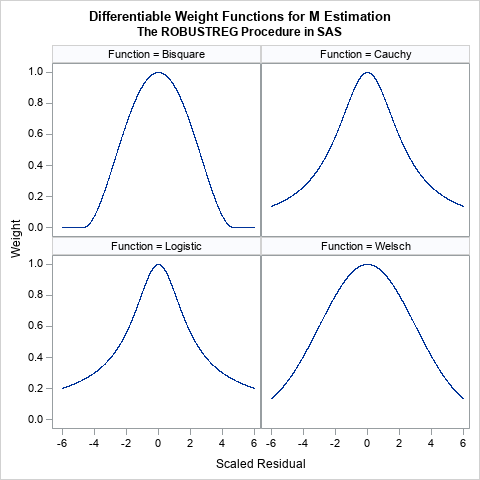These functions all look similar. They are all symmetric functions and look like the quadratic function 1 – a r2 close to r = 0. However, the functions differ in the rate at which they decrease away from r = 0:

• The bisquare function (also called the Tukey function) is a quartic function on the range [-c, c] and equals 0 outside that interval. This is the default weight function for M estimation in PROC ROBUSTREG. The function is chosen so that the derivative at ±c is 0, and thus the function is differentiable. An observation is excluded from the computation if the magnitude of the standardized residual exceeds c. By default, c=4.685.
• The Cauchy and Logistic functions are smooth functions that decrease exponentially. If you use these functions, the weight for an observation is never 0, but the weight falls off rapidly as the magnitude of r increases.
• The Welsch function is a rescaled Gaussian function. The weight for an observation is never 0, but the weight falls off rapidly (like exp(-a r2)) as the magnitude of r increases.

### Nondifferentiable weighting functions

The following six functions are not differentiable at one or more points.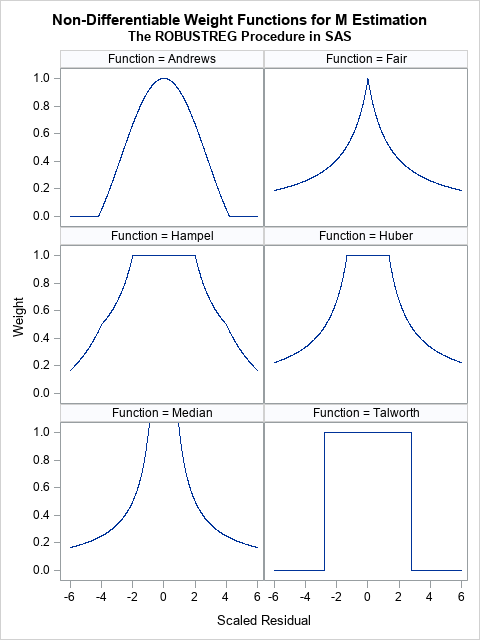• The Andrews function is the function c*sin(r/c)/r within the interval [-c π, c π] and 0 outside. a quartic function on the range [-c, c] and equals 0 outside that interval. It is similar to the bisquare function (see the previous section) but is not differentiable at ±c π.
• The Fair and Median functions are similar. The assign a weight that is asymptotically proportional to 1/|r|. Use the Fair function if you want to downweight observations according to the inverse magnitude of their residual value. Do not use the Median function, which assigns huge weights to observations with small residuals.
• The Hampel, Huber, and Talworth functions are similar because they all assign unit weight to residuals that are small in magnitude. The Talworth function is the most Draconian weight function: it assigns each observation a weight of 1 or 0, depending on whether the magnitude of the residual exceeds some threshold. The Hampel and Huber functions assign a weight of 1 for small residuals, then assign weights in a decreasing manner. If you use the default parameter values for the Hampel function, the weights are exactly 0 outside of the interval [-8, 8].

When I use M estimation, I typically use one of the following three functions:

• The bisquare function, which excludes observations whose residuals have a magnitude greater than 4.685.
• The Welsch function, which gently downweights large residuals.
• The Huber function, which assigns 1 for the weight of small residuals and assigns weights to larger residuals that are proportional to the inverse magnitude.

### Summary

M estimation is a robust regression technique that assigns weights to each observation. The idea is to assign small (or even zero) weights to observations that have large residuals and weights that are close to unity for residuals that are small. (In practice, the algorithm uses standardize residuals to assign weights.) There are many ways to assign weights, and SAS provides 10 different weighting functions. This article visualizes the weighting functions. The results of the regression depend on the function that you use to weight the residuals.

### Appendix: SAS program to visualize the weighting functions in PROC ROBUSTREG

I used SAS/IML to define the weighting functions because the language supports default arguments for functions, whereas PROC FCMP does not. Each function evaluates a vector of input values (x) and returns a vector of values for the weighting function. The default parameter values are specified by using the ARG=value notation in the function signature. If you do not specify the optional parameter, the default value is used.

The following statements produce a 2 x 2 panel of graphs that shows the shapes of the bisquare, Cauchy, logistic, and Welsch functions:

/***********************************/ /* Differentiable weight functions */ /* Assume x is a column vector */ /***********************************/   proc iml; /* The derivarive of Bisquare is f'(x)=4x(x^2-c^2)/c^4, so f'( +/-c )= 0 However, second derivative is f''(x) = 4(3x^2-c^2)/c^4, so f''(c) ^= 0 */ start Bisquare(x, c=4.685); /* Tukey's function */ w = j(nrow(x), 1, 0); /* initialize output */ idx = loc( abs(x) <= c ); if ncol(idx)>0 then do; y = x[idx]/c; w[idx] = (1 - y##2)##2; end; return ( w ); finish;   start Cauchy(x, c=2.385); w = 1 / (1 + (abs(x)/c)##2); return ( w ); finish;   start Logistic(x, c=1.205); w = j(nrow(x), 1, 1); /* initialize output */ idx = loc( abs(x) > constant('maceps') ); /* lim x->0 f(x) = 1 */ if ncol(idx)>0 then do; y = x[idx]/c; w[idx] = tanh(y) / y; end; return ( w ); finish;   start Welsch(x, c=2.985); w = exp( -(x/c)##2 / 2 ); return ( w ); finish;   x = T(do(-6, 6, 0.005)); Function = j(nrow(x), 1, BlankStr(12));   create WtFuncs var {'Function' 'x' 'y'}; y = Bisquare(x); Function[,] = "Bisquare"; append; y = Cauchy(x); Function[,] = "Cauchy"; append; y = Logistic(x); Function[,] = "Logistic"; append; y = Welsch(x); Function[,] = "Welsch"; append; close;   quit;   ods graphics/ width=480px height=480px; title "Differentiable Weight Functions for M Estimation"; title2 "The ROBUSTREG Procedure in SAS"; proc sgpanel data=WtFuncs; label x = "Scaled Residual" y="Weight"; panelby Function / columns=2; series x=x y=y; run;

The following statements produce a 3 x 2 panel of graphs that shows the shapes of the Andrews, Fair, Hampel, Huber, median, and Talworth functions:

/*******************************/ /* Non-smooth weight functions */ /* Assume x is a column vector */ /*******************************/ proc iml;   start Andrews(x, c=1.339); w = j(nrow(x), 1, 0); /* initialize output */ idx = loc( abs(x) <= constant('pi')*c ); if ncol(idx)>0 then do; y = x[idx]/c; w[idx] = sin(y) / y; end; return ( w ); finish;   start Fair(x, c=1.4); w = 1 / (1 + abs(x)/c); return ( w ); finish;   start Hampel(x, a=2, b=4, c=8); w = j(nrow(x), 1, 0); /* initialize output */ idx = loc( abs(x) <= a ); if ncol(idx)>0 then w[idx] = 1; idx = loc( a < abs(x) & abs(x) <= b ); if ncol(idx)>0 then w[idx] = a / abs(x[idx]); idx = loc( b < abs(x) & abs(x) <= c ); if ncol(idx)>0 then w[idx] = (a / abs(x[idx])) # (c - abs(x[idx])) / (c-b); return ( w ); finish;   start Huber(x, c=1.345); w = j(nrow(x), 1, 1); /* initialize output */ idx = loc( abs(x) >= c ); if ncol(idx)>0 then do; w[idx] = c / abs(x[idx]); end; return ( w ); finish;   start MedianWt(x, c=0.01); w = j(nrow(x), 1, 1/c); /* initialize output */ idx = loc( x ^= c ); if ncol(idx)>0 then do; w[idx] = 1 / abs(x[idx]); end; return ( w ); finish;   start Talworth(x, c=2.795); w = j(nrow(x), 1, 0); /* initialize output */ idx = loc( abs(x) < c ); if ncol(idx)>0 then do; w[idx] = 1; end; return ( w ); finish;   x = T(do(-6, 6, 0.01)); Function = j(nrow(x), 1, BlankStr(12)); create WtFuncs var {'Function' 'x' 'y'};   y = Andrews(x); Function[,] = "Andrews"; append; y = Fair(x); Function[,] = "Fair"; append; y = Hampel(x); Function[,] = "Hampel"; append; y = Huber(x); Function[,] = "Huber"; append; y = MedianWt(x); Function[,] = "Median"; append; y = Talworth(x); Function[,] = "Talworth";append; close; quit;   ods graphics/ width=480px height=640px; title "Non-Differentiable Weight Functions for M Estimation"; title2 "The ROBUSTREG Procedure in SAS"; proc sgpanel data=WtFuncs; label x = "Scaled Residual" y="Weight"; panelby Function / columns=2; series x=x y=y; rowaxis max=1; run;

The post Weights for residuals in robust regression appeared first on The DO Loop.

A common question on SAS discussion forums is how to randomly assign observations to groups. An application of this problem is assigning patients to cohorts in a clinical trial. For example, you might have 137 patients that you want to randomly assign to three groups: a control group, a group that gets an established treatment, and a group that gets a new treatment. Random assignment tends to produce groups in which patients who have confounding factors (obesity, high blood pressure, and other pre-existing conditions) are distributed equally across the groups.

There are many ways to perform a random assignment in SAS, including the DATA step and PROC PLAN. However, my favorite procedure for random assignment is to use the GROUPS= option in PROC SURVEYSELECT. This article shows three ways to assign subjects to groups:

• Assign the subjects as evenly as possible to G groups.
• Assign the subjects within each stratum as evenly as possible to G groups.
• Assign the subjects to groups by using a specified list of sample sizes.

### Distribute subjects equally among groups

In the simplest situation, you want to randomly assign N subjects to G groups so that the group sizes are as equal as possible. If G divides N, you can make the groups equal in size. Otherwise, one or more groups will have one more subject than the others.

Suppose that you have N=137 patients to assign to G=3 groups. Because 137 is a prime number, the groups cannot be equal. The following DATA step extracts 137 patients from the Sashelp.Heart data set. The call to PROC SURVEYSELECT uses the GROUP=3 option to randomly assign the patients to three groups. The output data set contains a new indicator variable that is named GroupID and has the values 1, 2, or 3. The call to PROC FREQ shows that the subjects are (nearly) equally divided among the three groups:

/* Extract 137 patients from the Sashelp.Heart dat set */ data Have; ID + 1; set Sashelp.Heart(obs=137); keep ID Sex Height Weight Diastolic Systolic Cholesterol; run;   /* SAS/STAT 13.1 : use GROUPS= option */ /* See https://support.sas.com/kb/36/383.html */ proc surveyselect data=Have noprint seed=12345 out=Cohorts groups=3; run;   proc freq data=Cohorts; table GroupID / nocum; /* count how many subjects in each group */ run;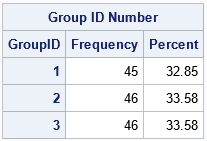The output shows that each group contains approximately 33% of the subjects. Forty-five subjects are assigned to the first group and 46 subjects are assigned to the second and third groups. When the number of groups does not evenly divide the number of subjects, the first groups have fewer subjects than the last groups.

As stated earlier, a benefit of random assignment is that covariate values have nearly identical distributions in each group. For example, the following statements create a box plot of the Cholesterol variable. The distribution of Cholesterol values seems to be similar in the three groups.

proc sgplot data=Cohorts; hbox Cholesterol / category=GroupID; run;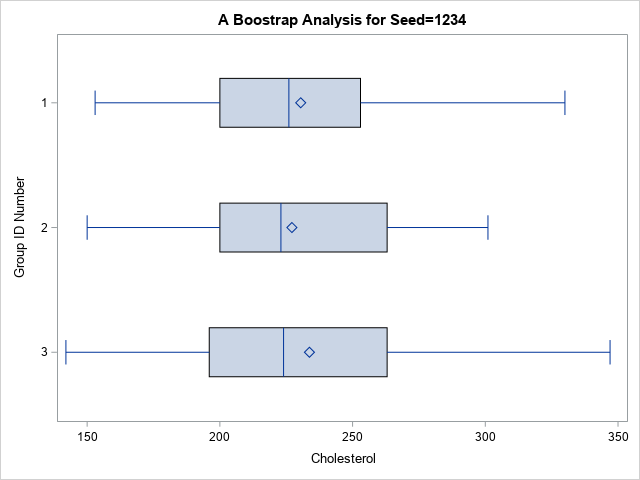### Distribute subjects within strata equally among groups

Sometimes the subjects have additional categorical characteristics such as Sex or Race. You might want to randomly assign subjects within each category. For example, these data contain 86 females and 51 males. The following call to PROC SURVEYSELECT uses the STRATA statement to separately assign the females to three groups (sizes are 28, 29, and 29) and the males to three groups (sizes are 17). To use the STRATA statement, you need to sort the data by the Sex variable, as follows:

/* random assignment within strata */ proc sort data=Have; by Sex; run;   proc surveyselect data=Have noprint seed=12345 out=Cohorts groups=3; strata Sex; run;   proc freq data=Cohorts; table Sex*GroupID / nocum nocol nopercent; run;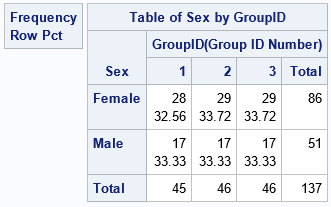The output from PROC FREQ shows that the random assignment is as even as possible within the males and within the females, separately, with about 33% of each gender in each group.

### Assign subjects unequally to groups

In some situations, you might not want an equal number of subjects in each group. For example, you might want to assign fewer subjects to the control group and more to the experimental groups. The GROUP= option supports a list of sample sizes. The following call to PROC SURVEYSELECT randomly assigns 40 subjects to each of the first two groups and 57 subjects to the third group:

/* You can also specify the groups sizes */ proc surveyselect data=Have noprint seed=12345 out=CohortSize groups=(40 40 57); run; proc freq data=CohortSize; table GroupID / nocum; run;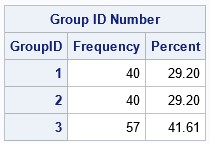The output from PROC FREQ verifies that the sample sizes for the three groups are as specified on the GROUP= list.

### Summary

This article shows that it is easy to use PROC SURVEYSELECT to carry out a random assignment of subjects to groups. This article shows three examples:

• Random assignment (evenly) to G groups.
• Random assignment within each stratum (evenly) to G groups.
• Random assignment to G groups when you want to specify the size of each group.